Thermodynamics and Propulsion

# 15.1 Fuels

There are a wide variety of fuels used for aerospace power and propulsion. A primary one is jet fuel, essentially kerosene. Kerosene is a mixture of various hydrocarbons. The fuels we consider here are hydrogen (), methane (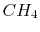), and octane (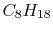).

The chemical process in which a fuel, for example methane, is burned consists of (on a very basic level -— there are many intermediate reactions that need to be accounted for when computations of the combustion process are carried out):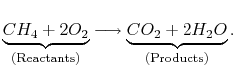The reactions we describe are carried out in air, which can be approximated as 21%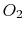and 79%. This composition is referred to as theoretical air.'' There are other components of air (for example Argon, which is roughly 1%), but the results given using the theoretical air approximation are more than adequate for our purposes. With this definition, for each mole of, 3.76 (or) moles ofare involved: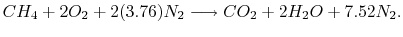Even if the nitrogen is not part of the combustion process, it leaves the combustion chamber at the same temperature as the other products, and this change in state (change in enthalpy) needs to be accounted for in the steady flow energy equation. At the high temperatures achieved in internal combustion engines (aircraft and automobile) reaction does occur between the nitrogen and oxygen, which gives rise to oxides of nitrogen, although we will not consider these reactions.

The condition at which the mixture of fuel and air is such that both completely participate in the reaction is called stoichiometric. In gas turbines, excess air is often used so that the temperatures of the gas exiting the combustor is kept to within desired limits (see Figures 3.20, 3.21, and 3.24(b) for data on these limits.)

Muddy Points

Why is there 3.76? (MP 15.1)

What is the most effective way to solve for the number of moles in the reactions? (MP 15.2)

UnifiedTP